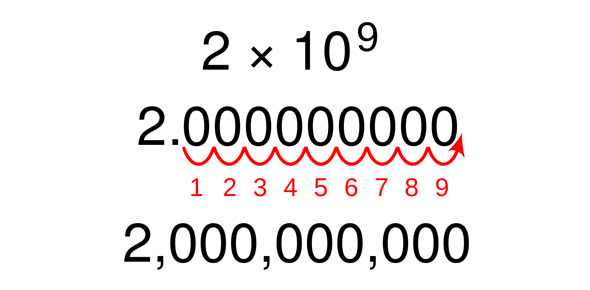# Scientific Notation: Multiplication, Division, Addition And Subtraction

10 Questions | Total Attempts: 3146SettingsPerforming mathematical computations with scientific notation.

• 1.
Is the following numbers in proper scientific notation? 23.2 x 102
• A.

Yes, this is proper scientific notation

• B.

No, this is not proper scientific notation

• 2.
Do NOT use your calculator. UNDERSTAND how to work with scientific notation!(1012 is the same as 10^12)(3.0x 107) (4.0 x 105)  =
• A.

12.0 x 10^12

• B.

12.0 x 10^35

• C.

1.2 x 10^13

• D.

1.2 x 10^36

• 3.
Find the answer:  9.0 x 103                          3.0 x 102
• A.

3.0 x 10^5

• B.

3.0 x 10^6

• C.

3.0 x 10^1

• D.

3

• E.

3.0 x 10^-1

• 4.
(8.0 x 10-2)  x  (3 x 10-3)  =
• A.

2.4 x 10^-5

• B.

24.0 x 10^-5

• C.

24.0 x 10^-6

• D.

2.4 x 10^-4

• 5.
(3.0 x 102) + (4.0 x 103) =
• A.

4.3 x 10^2

• B.

7.0 x 10^-1

• C.

4.3 x 10^3

• D.

7.0 x 10^5

• 6.
(9.0 x 103)  -  (4.0 x 102)
• A.

5.0 x 10^1

• B.

13.0 x 10^5

• C.

9.4 x 10^3

• D.

5.0 x 10^5

• E.

8.6 x 10^3

• 7.
(6.0 x 102) x (5.0 x 104)  =              (2.0 x 10 3)
• A.

1.5 x 10^4

• B.

1.5 x 10^8

• C.

15 x 10^4

• D.

6.0 x 10^4

• 8.
(6.0 x 102) + (4.0 x 104) =            (2.0 x 102)
• A.

5.0 x 10^4

• B.

2.03 x 10^2

• C.

1.2 x 10^8

• D.

5.0 x 10^5

• 9.
(8.0 x 10-2)   =  (2 x 10-3)
• A.

2 x 10^1 = 20

• B.

4 x 10^1 = 40

• C.

4 x 10^-5

• D.

4.0 x 10^-1

• 10.
What is this equivalent to? 6.2 x 10-3
• A.

.062

• B.

.62

• C.

0.0062

• D.

6,200

Related TopicsBack to top